# 决策树的原理及Python实现（一）ID3篇## 决策树的简介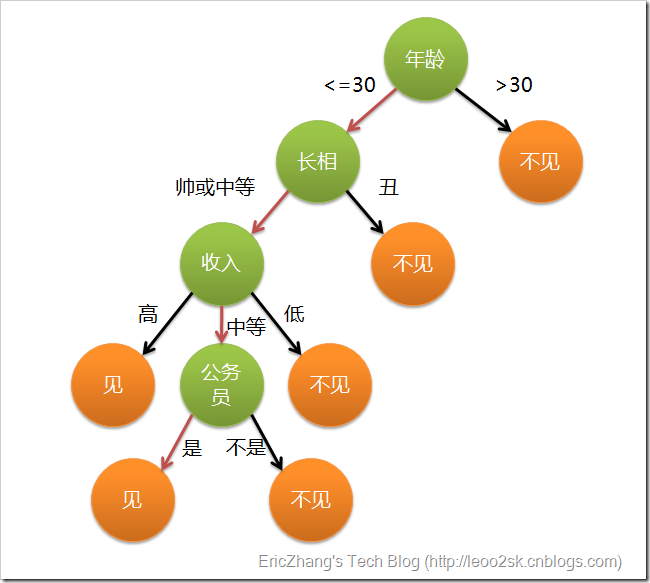## 决策树的分类

• 分类树 输出的结果是样本的类别，是离散值
• 回归树 输出的结果是一个实数，是连续值

• ID3算法：Iterative Dichotomiser 3，由Ross Quinlan发明，以信息增益度量属性选择
• C4.5算法：原理类似ID3算法，不同的是属性选择使用的是信息增益率
• CART算法：Classification And Regression Tree，决策树的一种，以GINI指数作为度量数据，采用二分递归分割生成二叉树

## ID3算法

ID3算法本质上是使用信息增益作为树分区划分的属性度量条件。 那么，什么是信息增益？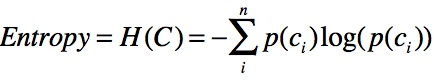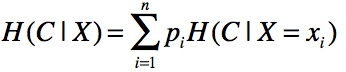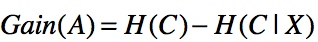### 一个示例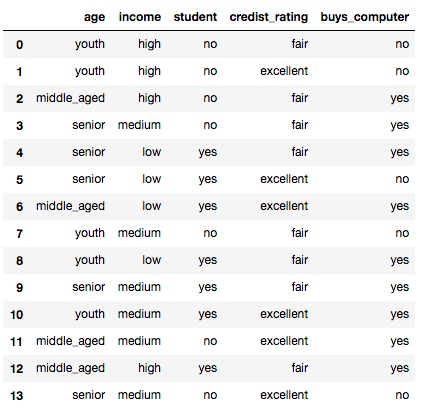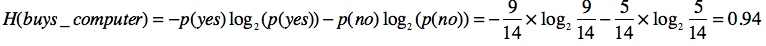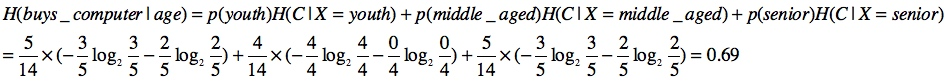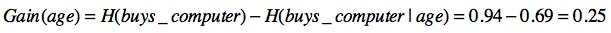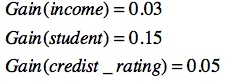### Python实现

import pandas as pd
from math import log
from anytree import Node, RenderTree
from anytree.dotexport import RenderTreeGraph

def create_decision_tree_id3(df, y_col):
# 计算H(C)
def h_value():
h = 0
for v in df.groupby(y_col).size().div(len(df)):
h += -v * log(v, 2)

return h

# 计算某一个属性的信息增益
def get_info_gain_byc(column, df, y_col):
# 计算p(column)
probs = df.groupby(column).size().div(len(df))
v = 0
for index1, v1 in probs.iteritems():
tmp_df = df[df[column] == index1]
tmp_probs = tmp_df.groupby(y_col).size().div(len(tmp_df))
tmp_v = 0
for v2 in tmp_probs:
# 计算H(C|X=xi)
tmp_v += -v2 * log(v2, 2)
# 计算H(y_col|column)
v += v1 * tmp_v
return v

# 获取拥有最大信息增益的属性
def get_max_info_gain(df, y_col):
d = {}
h = h_value()
for c in filter(lambda c: c != y_col, df.columns):
# 计算H(y_col) - H(y_col|column)
d[c] = h - get_info_gain_byc(c, df, y_col)

return max(d, key=d.get)

# 生成决策树
def train_decision_tree(node, df, y_col):
c = get_max_info_gain(df, y_col)
for v in pd.unique(df[c]):
gb = df[df[c] == v].groupby(y_col)
curr_node = Node('%s-%s' % (c, v), parent=node)
# 如果属性没有用完
if len(df.columns) > 2:
# 如果分区纯度是100%，则生成类别叶子节点
if len(gb) == 1:
Node(df[df[c] == v].groupby(c)[y_col].first().iloc, parent=curr_node)
else:
# 如果分区不纯则继续递归
train_decision_tree(curr_node, df[df[c] == v].drop(c, axis=1), y_col)
# 如果属性用完，则选择数量最多的类别实例作为类别叶子结点
else:
Node(df[df[c] == v].groupby(y_col).size().idxmax(), parent=curr_node)

root_node = Node('root')
train_decision_tree(root_node, df, y_col)
return root_node

for pre, fill, node in RenderTree(root_node):
print("%s%s" % (pre, node.name))

RenderTreeGraph(root_node).to_picture("decision_tree_id3.png")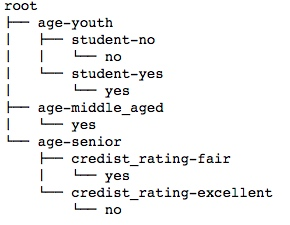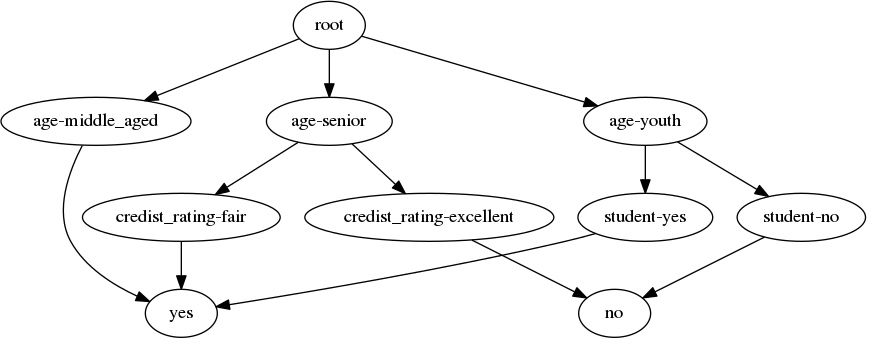• http://hunch.net/~coms-4771/quinlan.pdf